# JP2009211458A - Compiler, variable-optimizing device, method, and program - Google Patents

## Info

Publication number
JP2009211458A
JP2009211458A JP2008054361A JP2008054361A JP2009211458A JP 2009211458 A JP2009211458 A JP 2009211458A JP 2008054361 A JP2008054361 A JP 2008054361A JP 2008054361 A JP2008054361 A JP 2008054361A JP 2009211458 A JP2009211458 A JP 2009211458A
Authority
JP
Japan
Prior art keywords
array
code
sequence
expression
variable
Prior art date
Legal status (The legal status is an assumption and is not a legal conclusion. Google has not performed a legal analysis and makes no representation as to the accuracy of the status listed.)
Granted
Application number
JP2008054361A
Other languages
Japanese (ja)
Other versions
JP5169322B2 (en
Inventor
Yasuko Tatai

Original Assignee
Nec Corp

Priority date (The priority date is an assumption and is not a legal conclusion. Google has not performed a legal analysis and makes no representation as to the accuracy of the date listed.)
Filing date
Publication date
Application filed by Nec Corp, 日本電気株式会社 filed Critical Nec Corp
Priority to JP2008054361A priority Critical patent/JP5169322B2/en
Publication of JP2009211458A publication Critical patent/JP2009211458A/en
Application granted granted Critical
Publication of JP5169322B2 publication Critical patent/JP5169322B2/en
Active legal-status Critical Current
Anticipated expiration legal-status Critical

• 230000014509 gene expression Effects 0.000 claims abstract description 292
• 238000005457 optimization Methods 0.000 claims abstract description 133
• 238000000034 methods Methods 0.000 claims description 44
• 230000000875 corresponding Effects 0.000 claims description 16
• 238000006467 substitution reactions Methods 0.000 claims description 9
• 230000004927 fusion Effects 0.000 abstract description 6
• 241000212893 Chelon labrosus Species 0.000 description 9
• 238000007796 conventional methods Methods 0.000 description 2
• 238000010586 diagrams Methods 0.000 description 2
• 230000000052 comparative effects Effects 0.000 description 1
• 230000004048 modification Effects 0.000 description 1
• 238000006011 modification reactions Methods 0.000 description 1
• 230000001131 transforming Effects 0.000 description 1

## Images

•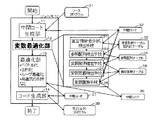••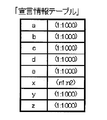•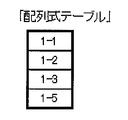•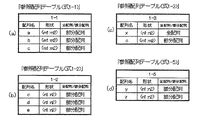•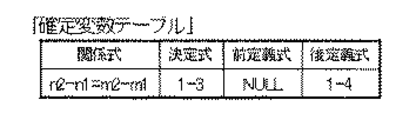••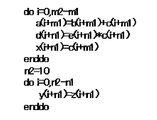•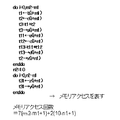•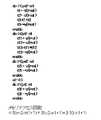•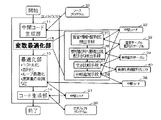••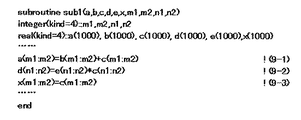•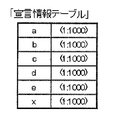••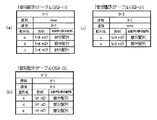•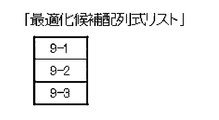••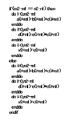•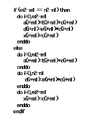••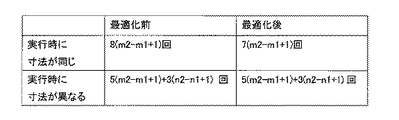•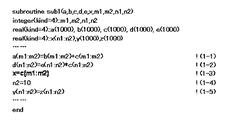•## Abstract

A variable optimization device capable of loop fusion even when the number of loops is defined using different variables and expressions.
Declaration information / array expression detection means 21 receives intermediate code 32, detects array declaration information and array expressions in a source program 31, and registers them in a declaration information table and array expression table 33. The reference sequence detection unit 22 refers to the declaration information table and the sequence expression table 33 to check the sequence referred to by the array formula, and registers the type of each sequence and the shape expressed by the lower limit / upper limit in the reference sequence table. . The variable relationship determining means 23 uses the fact that the dimensions of the arrays referenced by the same array formula are the same as each other to make the variable defining the lower limit / upper limit constant. Or, a relational expression between variables is determined. The variable replacement unit 25 replaces a variable included in the intermediate code by using a constant value or a determined relational expression.
[Selection] Figure 1

## Description

The present invention relates to a compiler and a variable optimization apparatus, and more particularly, a compiler that generates intermediate code from a source program and generates an object program from the intermediate code, and a variable optimization apparatus used for such a compiler. About. The present invention also relates to a compilation method, a variable optimization method, and a program.

In recent years, the operating speed of CPUs (processors) has been increasing due to improvements in hardware and the like. On the other hand, the performance of memory access has not been improved compared to the increase in CPU speed. For this reason, a program with little memory access can greatly improve the performance, but a program with many memory accesses has a small range of performance improvement. When generating a program, how to improve the performance of a program with many memory accesses is an issue.

The number of memory accesses can be reduced by performing loop transformation such as loop fusion, and by deleting the common expression to combine accesses to the same memory existing in a plurality of loops into one. By performing such processing in the compiler and reducing the number of memory accesses in the execution program, it is possible to improve performance in terms of software.

In a program such as Fortran, the lower limit / upper limit of an array referred to by an array formula may be declared using a variable. In that case, since the shape of the sequence cannot be determined at the time of translation, the sequence formula may not be sufficiently optimized. An example of a technique for compiling by replacing variables with constants is described in Patent Document 1. In Patent Document 1, a variable that has been defined once is selected, and the variable is replaced with a constant.
JP-A-2-118732

By the way, programming languages such as Fortran, which are widely used in scientific and engineering calculations, can calculate for each element of an array by describing the calculation of the array and the array, and the expression that assigns the calculation result in one sentence. An array expression is defined. FIG. 23 shows an example of a source program. A (m1: m2) in Formula 1-1 represents a partial array that is a part of the array including elements a (m1) to a (m2) of the array a. m1 is called the lower limit, and m2 is called the upper limit. The dimension of the array represents the number of elements of the array to be accessed. For example, in the case of a (1: 5), since the five elements a (1), a (2), a (3), a (4), and a (5) are included, the size of the array is 5. . In the expression 1-1, for elements from m1 to m2, an array b and an array c are expressed as a (m1) = b (m1) + c (m1) and a (m1 + 1) = b (m1 + 1) + c (m1 + 1). This means that the addition result for each element is assigned to each element of the array a. Since the calculation is performed for each element of the array, it is normal to describe only arrays of the same shape in one array formula. On the other hand, Formula 1-3 is the entire sequence. Expression 1-3 represents substituting each element of the partial array of c for each element of x.

The compiler internally generates a loop having an initial value and a final value calculated based on the lower limit and upper limit of the array appearing in the array expression. The compiler optimizes the generated loop, fuses the same number of loops, and deletes common expressions to reduce access to the same array elements in the loop and speed up the program. Plan. In order to fuse a plurality of loops in this optimization process, the number of loop iterations calculated based on the initial value and the final value of the loop must be the same during translation. However, in programs such as Fortran, the lower and upper bounds of an array referenced in an array expression may be declared using variables. In this case, the shape of the array cannot be determined during translation. In some cases, optimization could not be performed sufficiently. This will be described below.

FIG. 24 shows intermediate code generated from the source program of FIG. The array formulas of Formula 1-1 to Formula 1-3 and Formula 1-5 included in the source program of FIG. 23 are converted into four loops (from do to enddo) included in FIG. 24 by the intermediate code generation unit. The When converting to a loop, if the array is an entire array, the initial and final values of the loop are determined based on the lower and upper limits in the declaration expression, and in the case of a partial array, based on the lower and upper limits of the reference location. To determine an initial value and a closing price. For example, since the array formula 1-1 is an operation of the partial array (m1: m2) and the size is m2-m1 + 1, and the array formula 1-3 includes the partial array c (m1: m2). The initial value of the loop is set to 0 and the closing value m2-m1.

In the conventional method, using the fact that the dimensions and dimensions of all the arrays referenced in one array formula are the same, based on the lower limit and upper limit of one array referenced in the array formula, The initial value and the closing price were determined. For example, with regard to the array formula 1-3, the loop count is determined as m2-m1 + 1 by paying attention only to the number of dimensions and dimensions of the array referred to in the array formula 1-3. As described above, in the conventional method, the initial value and the final value of the loop are not determined based on the information on the lower limit / upper limit of all the arrays referenced in the array formula. If so, multiple sequence loops could not be fused between multiple sequence formulas. As a result, access to the same array element in the loop cannot be consolidated by deleting the common expression, and memory access cannot be reduced.

For example, in FIG. 24, the loop count of the array formulas 1-1 and 1-3 is set to m2-m1 + 1 times, and the loop count of the array formula 1-2 is set to n2-n1 + 1 times. Here, paying attention to the array formula 1-3, it can be seen that n2-n1 and m2-m1 are equal since the dimensions of the entire array x and the dimensions of the partial array c (m1: m2) are the same. If this information can be applied to other array formulas, the loop count n2-n1 + 1 in the array formula 1-2 can be replaced with m2-m1 + 1, and three loops can be combined into one. is there. However, in the prior art, since such an analysis is not performed, the compiler cannot recognize that m2-m1 and n2-n1 have the same value, and cannot compile a loop.

Regarding replacement of a variable with a constant, Patent Document 1 selects a variable that has been defined once and performs replacement from the variable to the constant. However, in Patent Document 1, a variable that is not defined in the procedure cannot be replaced with a constant. In addition, only variables are replaced with constants, and variables and expressions are not analyzed. For this reason, when the number of loops is defined using different variables or expressions, it is impossible to recognize that the number of loops is the same and fuse the loops.

The present invention provides a compiler, a variable optimization device, a method, and a program that can solve the above-described problems of the prior art and can perform loop fusion even when the number of loops is defined using different variables and expressions. The purpose is to do.

The variable optimization apparatus of the present invention inputs intermediate code based on a source program including an array expression, detects the declaration information of the array in the source program and the array expression, and registers them in the declaration information table and the array expression table Declaration information / array expression detection unit to be searched, the array referenced by the array expression by referring to the declaration information table and the array expression table, and the type of each array and the shape represented by the lower limit / upper limit are referred to as the reference array The lower limit / upper limit is defined by referring to the reference sequence detector registered in the table, the reference sequence table, and the declaration information table, and using the same dimension in each dimension of the sequence referenced in the same array formula. A variable relation determining unit that constants variables to be determined, or determines relational expressions between variables, and registers the constants or constant relations determined in a fixed variable table; Using the registration constants or relationship in Buru, the variables included in the intermediate code, characterized in that it comprises a variable replacement unit for replacing at the constant or equation.

The compiler according to the present invention detects an intermediate code generation unit that generates intermediate code based on a source program including an array expression, and array declaration information in the source program and the array expression based on the intermediate code. , A declaration information / array expression detection unit to be registered in the declaration information table and the array expression table, and the array referred to by the array expression with reference to the declaration information table and the array expression table, and the type of each array, the lower limit / A reference sequence detection unit that registers the shape represented by the upper limit in a reference sequence table, and the dimensions of each dimension of the sequences that are referred to by the same array formula are the same with reference to the reference sequence table and the declaration information table. The variable defining the lower limit / upper limit is made constant, or the relational expression between the variables is determined, and the constant or the determined relational expression is made into a definite variable table. A variable relation determining unit to be registered in the variable, a variable replacing unit for replacing a variable included in the intermediate code with the constant or relational expression, using the constant or relational expression registered in the fixed variable table, and the variable An optimization unit that performs at least loop optimization and deletion of common expressions on the intermediate code that has undergone variable substitution in the replacement unit, and outputs the processed intermediate code, and the intermediate code that is output by the optimization unit And a code generation unit for generating an object program.

The variable optimization method of the present invention is a method for performing optimization processing of variables in intermediate code based on a source program including an array formula using a computer, wherein the computer executes the source program based on the source program. Detecting the declaration information of the array and the array formula and registering them in the declaration information table and the array formula table, and the computer refers to the declaration information table and the array formula table in the array formula The sequence is examined, the type of each sequence and the shape represented by the lower limit / upper limit are registered in the reference sequence table, and the computer refers to the reference sequence table and the declaration information table and refers to the same sequence formula. The variable that defines the lower limit / upper limit is a constant using the fact that the dimension of each dimension of the array is the same Or determining a relational expression between variables and registering the constant or the determined relational expression in a fixed variable table; and the computer uses the constant or relational expression registered in the fixed variable table. And replacing a variable included in the intermediate code with the constant or the relational expression.

The compiling method of the present invention is a compiling method for compiling a source program including an array formula using a computer, wherein the computer generates intermediate code based on the source program including the array formula; Detecting the array declaration information in the source program and the array expression based on the intermediate code, and registering them in the declaration information table and the array expression table; and the computer includes the declaration information table and the array. A step of referring to an expression table to check an array to be referred to by the array expression, registering the type of each array and the shape represented by the lower limit / upper limit in the reference array table; and Refer to the declaration information table and measure the dimensions of each array in the same array formula. A step of making the variable defining the lower limit / upper limit constant, or determining a relational expression between variables, and registering the constant made constant or the determined relational expression in the definite variable table And the computer replaces a variable included in the intermediate code with the constant or relational expression using a constant or relational expression registered in the definite variable table; and the computer performs the replacement. A step of performing at least loop optimization and deletion of the common expression on the received intermediate code, outputting the processed intermediate code, and the computer generating an object program based on the processed intermediate code And a step.

A program of the present invention is a program that causes a computer to execute a variable optimization process in intermediate code based on a source program including an array formula, and that causes the computer to execute an array arrangement in the source program based on the source program. Declaration information and the array expression are detected and registered in the declaration information table and the array expression table, and the array referred to by the array expression is checked with reference to the declaration information table and the array expression table. The process of registering the type and the shape expressed by the lower limit / upper limit in the reference array table, and the dimensions of the dimensions of the arrays referenced by the same array formula are the same with reference to the reference array table and the declaration information table. The variable defining the lower limit / upper limit is made constant, or the relational expression between variables is determined and made constant. A process of registering a constant or a determined relational expression in the definite variable table and a process of replacing a variable included in the intermediate code with the constant or the relational expression using the constant or the relational expression registered in the definite variable table. Are executed.

A program according to the present invention is a program that causes a computer to compile a source program including an array formula, the computer generating an intermediate code based on the source program including the array formula, and the intermediate code Based on the declaration information of the array in the source program and the process of detecting the array expression and registering it in the declaration information table and the array expression table, and referring to the declaration information table and the array expression table, A process for checking the array to be referenced, registering the type of each array and the shape represented by the lower limit / upper limit in the reference array table, and referring to the reference array table and the declaration information table, and referring to the same array formula By making use of the fact that the dimensions of each dimension are the same, the variable defining the lower limit / upper limit is made constant or variable. A variable included in the intermediate code by using a process for determining a relational expression between them, registering the constant or the determined relational expression in a fixed variable table, and using the constant or the relational expression registered in the fixed variable table Is replaced with the constant or the relational expression, the intermediate code subjected to the replacement is at least loop optimized and the common expression is deleted, and the processed intermediate code is output, and the process Based on the later intermediate code, a process for generating an object program is executed.

The variable optimization apparatus of the present invention inputs intermediate code based on a source program including an array expression, detects the declaration information of the array in the source program and the array expression, and registers them in the declaration information table and the array expression table Declaration information / array expression detection unit to be searched, the array referred to by the array expression with reference to the declaration information table and the array expression table, the type of each array, the shape represented by the lower limit / upper limit, and the array The reference sequence detection unit that registers the sequence formula immediately before and after the formula in the reference sequence table, and the reference sequence table, the sequence size referenced by the sequence formula is the same during translation for the consecutive sequence formulas. If it is not possible to determine that they are the same, a size comparison unit that registers the consecutive sequence formulas in the optimization candidate sequence list, and the optimization candidate A branch addition unit for adding a code for selecting a case where the dimensions of consecutive array formulas are equal to each other and a case other than the dimensions of the array formulas registered in the array formula list to the intermediate code. And

The compiler according to the present invention detects an intermediate code generation unit that generates intermediate code based on a source program including an array expression, and array declaration information in the source program and the array expression based on the intermediate code. , A declaration information / array expression detection unit to be registered in the declaration information table and the array expression table, and the array referred to by the array expression with reference to the declaration information table and the array expression table, and the type of each array, the lower limit / A reference sequence detector that registers in the reference sequence table the shape represented by the upper limit and the sequence formula immediately before and after the sequence formula, and the reference sequence table. It is checked whether or not the size of the sequence to be referenced can be determined to be the same at the time of translation. A code for selecting between the dimension comparison unit to be registered in the list and the sequence formulas registered in the optimization candidate sequence list, when the dimensions of consecutive sequence formulas are equal to each other, A branch addition unit to be added to the code, and an optimization unit that performs at least loop optimization and deletion of the common expression on the intermediate code to which the code is added by the branch addition unit, and outputs the processed intermediate code And a code generation unit that generates an object program based on the intermediate code output from the optimization unit.

The variable optimization method of the present invention is a method for performing optimization processing of variables in intermediate code based on a source program including an array formula using a computer, wherein the computer executes the source program based on the intermediate code. And the step of detecting the array declaration information and the array formula and registering them in the declaration information table and the array formula table, and the computer refers to the declaration information table and the array formula table in the array formula Checking the array, registering in the reference sequence table the type of each sequence, the shape represented by the lower limit / upper limit, the sequence formula immediately before and after the sequence formula, and the computer, the reference sequence table Whether or not it is possible to determine that the dimensions of the sequences referenced in the sequence formula are the same during translation for consecutive sequence formulas If it is not possible to determine that they are identical, the step of registering the continuous sequence formulas in the optimization candidate sequence formula list, and the computer, for the sequence formulas registered in the optimization candidate sequence list, And a step of adding a code for selecting a case where the dimensions of the arrangement formulas to be equal to each other and a case other than the case are equal to each other to the intermediate code.

The compiling method of the present invention is a compiling method for compiling a source program including an array formula using a computer, in which the computer generates intermediate code based on the source program; Detecting the declaration information of the array in the source program and the array formula based on the intermediate code and registering them in the declaration information table and the array formula table; and the computer stores the declaration information table and the array formula table. A step of referring to the sequence referred to in the array formula and registering the type of each sequence, the shape represented by the lower limit / upper limit, and the sequence formula immediately before and after the sequence formula in the reference sequence table; , The computer refers to the reference sequence table, for a continuous sequence formula, Check whether or not it is possible to determine that the dimensions of the sequences referred to in the column formula are the same at the time of translation, and when it is not possible to determine that they are the same, registering the consecutive sequence formula in the optimization candidate sequence formula list; A step of adding, to the intermediate code, a code for selecting, when the sequence formulas registered in the optimization candidate sequence formula list, the dimensions of consecutive sequence formulas are equal to each other, and other cases; The computer performs at least loop optimization and common expression deletion on the intermediate code to which the code is added, and outputs the processed intermediate code; and the computer outputs the intermediate code after the processing. Generating an object program based on the code.

A program according to the present invention is a program that causes a computer to execute a variable optimization process in an intermediate code based on a source program including an array formula, and that causes the computer to execute an array process in the source program based on the intermediate code. Declaration information and the array expression are detected and registered in the declaration information table and the array expression table, and the array referred to by the array expression is checked with reference to the declaration information table and the array expression table. Processing for registering the type, the shape represented by the lower limit / upper limit, the array formula immediately before and after the array formula in the reference sequence table, and referring to the reference sequence table, Check whether the dimensions of the sequences referred to in the formula can be judged to be the same at the time of translation. A process of registering continuous sequence formulas in the optimization candidate sequence formula list, and a sequence formula registered in the optimization candidate sequence formula list, when the dimensions of the continuous sequence formulas are equal to each other, and in other cases And a process of adding a code for selecting to the intermediate code.

A program according to the present invention is a program for causing a computer to execute a process of compiling a source program including an array formula, wherein the computer generates an intermediate code based on the source program; Based on the declaration information of the array in the source program and the process of detecting the array expression and registering it in the declaration information table and the array expression table, and referring to the declaration information table and the array expression table, Check the sequence to be referenced, register the type of each sequence, the shape represented by the lower limit / upper limit, the sequence formula immediately before and after the sequence formula in the reference sequence table, and refer to the reference sequence table. Whether or not it is possible to determine that the dimensions of the sequences referred to in the sequence formula are the same during translation. When it is not possible to determine that they are identical, the process of registering the consecutive sequence formulas in the optimization candidate sequence list and the sequence formulas registered in the optimization candidate sequence list have dimensions of consecutive sequence formulas. A process of adding a code for selecting a case equal to each other and a case other than that to the intermediate code; and the computer performs at least loop optimization and a common expression for the intermediate code to which the code is added. It is characterized in that it has a process of deleting and outputting the processed intermediate code and a process of generating an object program based on the processed intermediate code.

In the compiler, variable optimization device, method, and program of the present invention, loop fusion is possible even when the number of loops is defined using different variables and expressions.

Hereinafter, embodiments of the present invention will be described in detail with reference to the drawings. FIG. 1 shows a compiler according to the first embodiment of the present invention. The compiler 10 includes an intermediate code generation unit 11, a variable optimization unit 12, an optimization unit 13, and a code generation unit 14. The compiler translates a program such as Fortran and generates a load module. The function of each part of the compiler can be realized by executing a predetermined program on a computer.

The source program 31 is a source of a program written in a predetermined programming language such as Fortran. The source program 31 is stored in a memory or the like. The intermediate code generation unit 11 reads the source program 31 and generates an intermediate code 32 based on the source program 31. The generated intermediate code 32 is stored in a memory or the like.

The variable optimization unit 12 reads the intermediate code 32, performs variable optimization processing in the intermediate code 32, and generates an intermediate code 36. The generated intermediate code 36 is stored in a memory or the like. The optimization unit 13 performs vectorization, parallelization, loop optimization, common expression deletion, and the like on the intermediate code 36. The processing performed by the optimizing unit 13 includes at least loop optimization for grouping loops having the same number of loops and deletion of common expressions. The optimization unit 13 stores the intermediate code 37 as a result of the loop optimization or the like in a memory or the like.

The code generation unit 14 reads the intermediate code 37 optimized by the optimization unit 13 and generates an object program 38 based on the intermediate code 37. The generated object program 38 is stored in a memory or a holding storage device. As the intermediate code generation unit 11, the optimization unit 13, and the code generation unit 14, existing intermediate code generation means, optimization means, and code generation means can be used, respectively.

The variable optimization unit 12 includes declaration information / array expression detection means 21, reference array detection means 22, variable relationship determination means 23, variable definition detection means 24, and variable replacement means 25. The declaration information / array expression detection means 21 searches the intermediate code 32 to detect array declaration information and array expressions, and registers them in the declaration information table and array expression table 33. The reference sequence detection means 22 checks the sequence referred to in each sequence formula with respect to the sequence formula registered in the sequence formula table, and for each sequence, the type of sequence (full sequence / partial sequence), the lower limit of the sequence And the upper limit are registered in the reference sequence table 34. The upper limit and lower limit of the array are the lower limit and the upper limit registered in the declaration information table if the array is an entire array, and the lower limit and the upper limit at the reference location if the array is a partial array.

The variable relationship determining means 23 refers to the reference array table 34, calculates the dimensions of the array based on the lower limit and the upper limit of the array, and utilizes the fact that the dimensions of the arrays in the same array formula are the same. To determine the values of variables and formulas used to calculate dimensions. If the value is not determined, the relationship with other variables and expressions is determined. For example, when a sequence having a lower limit / upper limit of n1 / n2 and a sequence having a lower limit / upper limit of m1 / m2 are referred to in the same sequence formula, the fact that both dimensions are the same, The relational expression between them is determined as n2-n1 = m2-m1. The variable relationship determining unit 23 registers a relational expression representing the determined value or relationship and a determination expression that is an array expression that has determined the relation in the fixed variable table 35.

The variable definition detection unit 24 searches the intermediate code 32 before and after the determination formula registered in the fixed variable table 35 as a starting point, and the relational expression registered in the fixed variable table 35 is the lower limit or upper limit of the partial array. Check if it refers to a variable that appears. If they are referenced, search for the expression that defines the variable referenced by the relational expression before and after the decision expression, and define the variable that defines the variable before the decision expression (pre-defined expression) and the variable after it. And an expression (post-definition expression) to be detected. The variable definition detecting unit 24 registers the detected pre-defined formula and post-defined formula in the fixed variable table 35. When a plurality of pre-defined formulas and post-defined formulas are detected, a formula that may be executed in the execution path closest to the decision formula is registered. If there is no expression to be defined, NULL is registered in the pre-defined expression or post-defined expression of the definite variable table 35. When only the variables used in the declaration expressions of all the arrays are referred to, NULL is registered in the pre-defined expression and the post-defined expression of the defined variable table 35.

The variable replacement means 25 searches the intermediate code 32 and refers to the right side or the left side (hereinafter, described as the left side) of the relational expression registered in the defined variable table 35 between the pre-defined formula and the post-defined formula. A place to be detected is detected, and this is replaced with the right side of the relational expression. In the search, when the pre-defined formula is NULL, the search is performed from the beginning to the post-defined formula, and when the post-defined formula is NULL, the search is performed from the pre-defined formula to the end. For example, if the relational expression is n2-n1 = m2-m1, if n2-n1 exists between the pre-defined expression and the post-defined expression in the intermediate code 32, it is replaced with m2-m1. To do. The variable replacement unit 25 outputs the replacement result as an intermediate code 36.

The intermediate code 36 generated by the variable optimizing unit 12 is subjected to optimization such as loop optimization and common expression deletion in the optimizing unit 13, and memory access is reduced compared to the intermediate code 36. Intermediate code 37 is obtained. By generating the object program 38 from the intermediate code 37 in the code generation unit 14, the object program is generated from the intermediate code that has been optimized by the optimization unit 13 with respect to the intermediate code 32 generated by the intermediate code generation unit 11. As compared with the case of generating the program, a program with reduced memory access can be obtained.

FIG. 2 shows an operation procedure of the variable optimization unit 12. The declaration information / array expression detecting means 21 searches the intermediate code 32, detects the array declaration information and the array expression, and registers them in the declaration information table and the array expression table 33 (step S1). 3 and 4 show data examples of the declaration information table and the array formula table, respectively. By detecting the declaration information of the array from the intermediate code 32 corresponding to the source program 31 shown in FIG. 23, the arrays a, b, c, d, e, x, y, and z are registered in the declaration information table ( FIG. 3). Further, by detecting an array formula from the intermediate code 32, array formulas corresponding to the array formulas 1-1 to 1-3 and 1-5 of FIG. 23 are registered in the array formula table (FIG. 4).

The reference sequence detection means 22 creates a reference sequence table 34 for each sequence formula registered in the sequence formula table, and the array name and sequence of the sequence referenced by each sequence formula are stored in each reference sequence table. And the type of array (full array / partial array) are registered (step S2). For the shape (lower limit: upper limit), if the array is an entire array, the lower limit and the upper limit registered in the declaration information table are registered. If the array is a partial array, the lower limit and the upper limit at the reference location are registered. 5A to 5D show data examples of the reference sequence table. Referring to the array formula table shown in FIG. 4, there are four array formulas (array formulas 1-1 to 1-3 and 1-5) in the intermediate code 32, so a reference sequence table is created corresponding to these. (FIGS. 5A to 5D).

In step S2, arrays a, b, and c are set for expression 1-1 (FIG. 5A), and arrays c, d, and e are set for expression 1-2 (FIG. 5B), C and x are registered in the reference sequence table for Expression 1-3 (FIG. 5C), and y and z are stored for Expression 1-5 (FIG. 5D). In addition, the fact that the array is the entire array is registered for the array x in Formula 1-3, and the fact that it is a partial array is registered for the other arrays. For x, which is the entire array, the shape (n1: n2) registered in the declaration information table is registered in the reference array table 34, and for other arrays, the shape of the reference location is registered in the reference array table 34. For example, referring to FIG. 23, since the reference location of the array a in Expression 1-1 is m1 to m2, (m1: m2) is registered as the shape of the array a in the reference array table for Expression 1-1. To do.

The variable relationship determining means 23 refers to the reference sequence table 34, calculates the size from the lower limit and the upper limit of the array in each array formula, and utilizes the fact that the dimensions of each array in the same array formula are the same. Then, the values of variables and expressions to be referred to in the dimension calculation are determined. For example, in the same reference sequence table, when there are an array whose shape is defined by two variables and an array defined by two constants, using the fact that the dimensions of the arrays are the same, Variables can be made constant from the relationship. When the value is not determined, the relationship with other variables and expressions is determined. For example, in the same reference sequence table, when there is an array whose shape is defined by two variables and another array whose shape is defined by two different variables, the fact that both dimensions are the same is used. Thus, the relational expression between the four variables can be derived. The variable relationship determining unit 23 registers a relational expression representing the determined value or relationship and a determination expression that is an array expression that has determined the relation in the fixed variable table 35 (step S3).

FIG. 6 shows an example of data in the definite variable table 35. Referring to the reference sequence tables (FIGS. 5A, 5B, and 5D) corresponding to the expressions 1-1, 1-2, and 1-5, in these, variables (m1, m2, or n1 having the same shape) , N2), there is no value or relational expression that can be determined from these. Referring to the reference sequence table corresponding to Equation 1-3 (FIG. 5C), the shape of the array x is (n1: n2), and the shape (reference location) of the array c is (m1: m2). Yes. Using the fact that both shapes are equal, a relational expression of n1-n2 = m1-m2 is obtained. The variable relationship determining unit 23 registers the obtained relational expression “n1-n2 = m1-m2” and the array formula “1-3” for which the relational expression is determined in the fixed variable table 35.

The variable definition detection unit 24 searches the intermediate code 32 before and after the determination formula registered in the definite variable table 35, and refers to the variable in which the relational expression determined by the determination formula appears in the lower limit / upper limit of the partial array. Judge whether or not. If it is referenced, search for an expression that defines a variable referenced by the relational expression before and after the decision expression. The variable definition detection means 24 registers the expression that defines the variable before the decision formula obtained in the search as a pre-defined formula in the fixed variable table 35, and the formula that defines the variable after the decision formula is the post-defined formula. Is registered in the definite variable table 35 (step S4). When a plurality of pre-defined formulas or post-defined formulas are searched in the search, the formula that may be executed closest to the decision formula in the execution path is registered. If there are no pre-defined formula and post-defined formula, NULL is registered in the definite variable table 35. If the relational expression determined by the determination formula does not refer to a variable appearing at the lower limit / upper limit of the partial array, NULL is registered in the defined variable table 35.

Whether or not the relational expression “n2-n1 = m2-m1” determined by the determination formula “1-3” refers to a variable appearing at the lower limit / upper limit of the partial sequence in the source program shown in FIG. When examined, the relational expressions refer to variables appearing at the lower limit / upper limit of the partial array in the array formulas 1-1, 1-2, and 1-5. When an expression that defines a variable to be referred to by a relational expression is searched before and after the determination expression, the value of n2 is defined by Expression 1-4 after the determination expression. Does not exist to define before the determinant. Therefore, the variable definition detection unit 24 registers Formula 1-4 in the post-definition formula of the definite variable table 35 and registers NULL in the pre-definition formula.

If there is an expression that defines a variable of the relational expression before the decision expression, it is unclear whether the relation defined by the relational expression can be used as it is before the previous definition expression. Similarly, if there is an expression that defines a variable of a relational expression after the determination formula, it is unclear whether the relation defined by the relational expression can be applied after the definition formula. Therefore, the range in which the relational expression can be used is a range sandwiched between the pre-defined formula and the post-defined formula in the intermediate code 32. In FIG. 23, in the array formulas 1-1, 1-2, and 1-5, the relational expression refers to a variable that appears at the lower limit / upper limit of the partial array. Since it is after the post-defining formula, the relational formula can be applied to the array formulas 1-1 and 1-2.

The variable replacement unit 25 searches the intermediate code 32 while referring to the fixed variable table 35 and the reference array table 34, and the relational expression registered in the fixed variable table 35 between the pre-defined formula and the post-defined formula. A part corresponding to the left side is detected, and the part is replaced with the right side (step S5). If a value has been determined, replace it with that value. If the pre-defined formula is NULL, the search is performed in the range from the beginning of the intermediate code to the post-defined formula, and if the post-defined formula is NULL, the search is performed in the range from the pre-defined formula to the end of the intermediate code. The variable replacement means 25 outputs the intermediate code 36 after the replacement.

FIG. 7 shows the intermediate code 36 after the variable optimization unit 12 performs variable optimization. The intermediate code 32 before variable optimization is as shown in FIG. 24, and the array formulas 1-1 to 1-3 are independent loops. The number of loops is m2-m1 + 1 times, n2-n1 + 1 times, and m2-m1 + 1 times. In step S5, the relational expression “n2-n1 = m2-m1” is used to replace the portion represented by n2-n1 with m2-m1 in the intermediate code 32 in the range from the beginning to Expression 1-4. . Thereby, the intermediate code 36 shown in FIG. 7 is obtained.

FIG. 8 shows an intermediate code 37 obtained by performing loop optimization and common expression deletion on the intermediate code 36. The intermediate code 37 shown in FIG. 7 is obtained by performing the loop optimization and the deletion of the common expression in the optimization unit 13 to obtain the intermediate code 37 with reduced memory access (FIG. 8). That is, the intermediate code 37 in which the array formulas 1-1 to 1-3 are described in one loop is obtained. Thereafter, an object program 38 is generated by the code generation unit 14 for the intermediate code 37, so that a memory access is reduced and a high-speed program is obtained.

FIG. 9 shows the memory access and operation to be executed. As a comparative example, FIG. 10 shows memory access and calculation executed when an object program is generated from the intermediate code shown in FIG. In FIGS. 9 and 10, a portion indicated by an arrow represents a memory access. When the object program is generated from the intermediate code of FIG. 24, the number of memory accesses is 5 (m2-m1 + 1) +3 (n2-n1 + 1) +2 (10-n1 + 1) times. Since m2−m1 = n2−n1, this can be rewritten as 8 (m2−m1 + 1) +2 (10−n1 + 1). On the other hand, the number of memory accesses when the object program is generated from the intermediate code shown in FIG. 8 is 7 (m2−m1 + 1) +2 (10−n1 + 1) times. Therefore, by performing variable optimization processing, the number of memory accesses can be reduced by (m2−m1 + 1) times, and the program execution can be speeded up by that amount.

In the present embodiment, the variable relationship determination unit 23 defines the lower limit / upper limit of each array referred to in the array formula using the fact that the dimensions of the arrays constituting the same array formula are the same. Determine the value of a variable or expression, or determine the relational expression between variables. When the value is determined, the value of the variable used in the array declaration in the procedure can be determined if it is an entire array, and the value of the variable or expression that determines the reference location can be determined if it is a partial array. When the value is not determined, the relationship with other variables and expressions is determined. For the partial array, it is analyzed whether or not the determined value or relationship is changed by another expression, and a range in which the determined value or relationship can be applied is obtained. Thereafter, the variable substitution means 25 converts the variable or expression into a constant according to the determined value. Also, using the determined relational expression, the variable or expression is replaced with the same variable or the same expression.

The compiler internally generates a loop having an initial value and a final value calculated based on the lower limit and upper limit of the array appearing in the array expression. Whether or not the number of loops is the same since the initial value and the final value are defined in different variables by performing variable substitution in the variable substitution means 25 and making the variables constant and unifying the variables. It becomes possible to determine that the number of loops of a plurality of unknown loops is the same number of loops. As a result, loop fusion is possible and common expressions can be deleted. By deleting common expressions and grouping accesses to the same array element in a loop, the number of memory accesses can be reduced. Thereby, the program execution time can be shortened.

Next, a second embodiment of the present invention will be described. FIG. 11 shows a compiler according to the second embodiment of the present invention. In the compiler 10 a of this embodiment, the variable optimization unit 15 includes declaration information / array expression detection means 21, reference array / continuous appearance array expression detection means 26, dimension comparison means 27, and branch addition means 28. In the first embodiment, when the variable optimizing unit 12 (FIG. 1) determines a variable or expression value that is referred to in the calculation of dimensions of each dimension at the time of translation, or a variable or expression having the same value, Replaced the original expression with the determined variable or expression. In the present embodiment, when a variable or expression value that is referred to in the calculation of dimensions of each dimension at the time of translation, or a variable or expression having the same value is not determined, a code that selects a code to be executed at the time of execution is generated.

The declaration information / array expression detection means 21 searches the intermediate code 32, detects the upper and lower limits of the array declaration, and the array expression, and registers them in the declaration information table and the array expression table 33. The reference sequence / sequentially appearing sequence type detection means 26 is configured to select the sequence referenced in each sequence type, the type of sequence (total sequence / partial sequence), the lower limit of the sequence, and the sequence type registered in the sequence type table. The upper limit is checked and registered in the reference sequence table 39. Further, it is checked whether or not the array formula continues immediately before and after the array formula. If the array formula continues immediately before or immediately after, the array formula is registered in the reference sequence table 39.

The size comparison unit 27 refers to the reference sequence table 39 to check whether or not it is possible to determine that the size of the sequence referred to in the continuous sequence formula is the same during translation. If there is a continuous array formula that cannot be determined to have the same size at the time of translation, the array formula is registered in the optimization candidate sequence list 40. The branch addition means 28 generates an intermediate code 36 in which codes for selecting whether the dimensions are the same or not are added to the intermediate code 32 for the array formulas registered in the optimization candidate sequence list 40. To do. For the code executed when the dimensions are the same, the initial value and closing value of the other loop are replaced with the initial value and closing value of the loop generated for the first array expression.

FIG. 12 shows an operation procedure of the variable optimization unit 15 of this embodiment. The declaration information / array expression detecting means 21 searches the intermediate code 32, detects the array declaration information and the array expression, and registers them in the declaration information table and the array expression table 33 (step S11). FIG. 13 shows an example of a source program. 14 and 15 show data examples of the declaration information table and the array formula table 33. FIG. By detecting array declaration information from the intermediate code 32 corresponding to the source program 31 shown in FIG. 13, the arrays a, b, c, d, e, and x are registered in the declaration information table (FIG. 14). Further, by detecting the array formula from the intermediate code 32, the array formula corresponding to the array formulas 9-1 to 9-3 in FIG. 13 is registered in the array formula table (FIG. 15).

The reference sequence / continuous appearance sequence type detection means 26 creates a reference sequence table 39 for each sequence type registered in the sequence type table, and stores the reference sequence table 39 in each reference sequence table. The array name, array shape, and array type (full array / partial array) are registered. For each array formula, when there is an array formula immediately before or after the array formula, the array formula is registered in the reference sequence table 39 as the sequence formula immediately before or after (step S12). When registering the array shape (lower limit: upper limit), if the array is an entire array, register the lower limit and upper limit registered in the declaration information table, and if it is a partial array, register the lower limit and upper limit at the reference location. To do.

FIGS. 16A to 16C show data examples of the reference sequence table. Referring to the array formula table shown in FIG. 15, since there are three array formulas (array formulas 9-1 to 9-3) in the intermediate code, the reference sequence / continuous appearance array formula detection means 26 corresponds to these. Thus, a reference sequence table is created (FIGS. 16A to 16C). Thereafter, arrays a, b, and c are assigned to 9-1 (FIG. 16 (a)), and arrays c, d, and e are assigned to expression 9-2 (FIG. 16 (b)) and expressions 9-3. C and x are registered in the reference sequence table (FIG. 16C). The array types are all partial arrays. As the shape, the shape of the reference location of each array is registered in the reference array table 39. For example, referring to FIG. 13, since the reference location of the array a in Expression 9-1 is m1 to m2, (m1: m2) is registered as the shape of the array a in the reference array table for Expression 9-1. To do.

Referring to FIG. 13, there is no other array formula immediately before the array formula 9-1, and immediately after the array formula 9-2. The reference sequence / sequentially appearing sequence expression detection means 26 registers “none” indicating that there is no sequence expression in “just before” of the reference sequence table corresponding to the array formula 9-1, and immediately after that, the array formula 9− 2 is registered. As for the array formula 9-2, since there is the array formula 9-1 immediately before and there is the array formula 9-3 immediately after, the array formula 9-1 is registered “just before” in the reference sequence table, and “immediately after”. The array formula 9-3 is registered in. Since the array formula 9-3 has the array formula 9-2 immediately before and there is no array formula immediately after it, the array formula 9-2 is registered “immediately before” in the reference sequence table, and “one” is set to “none”. sign up.

The dimension comparison means 27 refers to the reference sequence table 39 and detects a location where the array formulas are continuous. Thereafter, for successive sequence formulas, it is examined whether or not the dimensions of the sequences referred to in these sequence formulas can be determined to be the same during translation. If there is a continuous array formula that cannot be determined to have the same dimension during translation, the array formula is registered in the optimization candidate sequence list 40 (step S13). Referring to FIGS. 16A to 16C, by examining “immediately before” and “immediately” of each array formula, it is detected that array formulas 9-1 to 9-3 are continuous array formulas. After detecting the consecutive array formulas, it is determined whether or not it is possible to determine that the dimensions of the arrays referred to in the continuous array formulas 9-1 to 9-3 are the same by referring to the shape of each array in the reference sequence table 39. Investigate.

FIG. 17 shows a data example of the optimization candidate sequence expression list 40. Referring to FIG. 16A, the size of the array referred to in the array formula 9-1 is m2-m1 + 1. Referring to FIG. 16B, the dimension of the array referred to in the array formula 9-2 is n2-n1 + 1. In this case, since it is unclear whether or not the size of the sequence to be referenced is the same between the array formula 9-1 and the array formula 9-2, the size comparison unit 27 includes the optimization candidate sequence formula list 40, The array formulas 9-1 and 9-2 are registered (FIG. 17). Similarly, when it is determined whether or not it is possible to determine that the size of the array to be referred to is the same in the array formula 9-2 and the array formula 9-3, the size of the array to be referred to in the array formula 9-3 is m2- Since it is m1 + 1, it cannot be judged that it is the same. The dimension comparison unit 27 additionally registers the sequence formula 9-3 in the optimization candidate sequence formula list 40.

The branch adding means 28 adds an intermediate code 36 to the intermediate code 32 by adding, to the intermediate code 32, a code for selecting whether the dimensions are the same or not for the array formulas registered in the optimization candidate sequence list 40. Is generated (step S14). The intermediate code 36 generated by the branch addition means 28 becomes the intermediate code output from the variable optimization unit 15. 18 and 19 show examples of intermediate code data before and after addition by the branch addition means 28. FIG. The intermediate code 32 shown in FIG. 18 is an intermediate code corresponding to the source program shown in FIG. 13 generated by the intermediate code generator 11. The branch addition means 28 generates a code to be executed from the intermediate code 32 when the dimensions of the arrays referred to in the array formulas 9-1 to 9-3 are the same, that is, m2-m1 = n2-n1 is established. Then, an intermediate code 36 to which this is added is generated (FIG. 19).

The intermediate code 36 shown in FIG. 19 includes a code part (between if and else) executed when m2-m1 = n2-n1 is established and a code part (between else and endif) executed when m2−m1 = n2−n1 is not established. ). The code portion executed when the dimensions are the same is obtained by replacing the initial value and the end value of the loop corresponding to each array expression in the intermediate code 32 (FIG. 18) as the generation source with the array expression 9-1 which is the first array expression. It is replaced with the initial value and the closing price of the corresponding loop. That is, in FIG. 18, the initial value and the closing price of the loop corresponding to the array formula 9-2 that had the initial value = 0 and the closing price n2−n1 are the initial value and the closing price of the loop corresponding to the array formula 9-1, respectively. 0 and m2-m1 are replaced. The contents of the code portion executed when the dimensions are not the same are the same as those shown in FIG.

The optimization unit 13 optimizes the intermediate code 36 (FIG. 19) output from the variable optimization unit 15. FIG. 20 shows the intermediate code 37 after optimization. In the optimized intermediate code 37, the loop is combined into one in the code portion that is executed when m2-m1 = n2-n1 holds. On the other hand, the code portion executed when m2-m1 = n2-n1 is not established remains the same as the intermediate code 32 shown in FIG. 18 because loop fusion cannot be performed.

FIG. 21 shows memory access and calculation when the intermediate code shown in FIG. 20 is executed. When the object program generated based on the optimized intermediate code 37 is executed, the number of memory accesses when m2-m1 = n2-n1 is satisfied at the time of execution is 7 (m2-m1 + 1) times. Further, the number of memory accesses when m2−m1 = n2−n1 is not satisfied at the time of execution is 5 (m2−m1 + 1) +3 (n2−n1 + 1) times.

When the intermediate code 32 shown in FIG. 18 is optimized by the optimization unit 13 without passing through the variable optimization unit 15, the code executed when m2-m1 = n2-n1 in the intermediate code 36 is not satisfied. Like the part, there is no place where the loop is fused. Therefore, when the object program generated based on the intermediate code 32 is executed, the number of memory accesses is 5 (m2−m1 + 1) +3 (n2−n1 + 1). When the values of m2-m1 and n2-n1 are equal, the number of memory accesses can be expressed as 8 (m2-m1 + 1) times.

FIG. 22 shows a comparison table of the number of memory accesses. When the object program based on the intermediate code 36 is executed, the number of memory accesses is the same, that is, the size is different 7 times (m2−m1 + 1) when m2−m1 = n2−n1 holds, that is, m2. When -m1 = n2-n1 is not satisfied, 5 (m2-m1 + 1) +3 (n2-n1 + 1) is obtained. On the other hand, when the object program based on the intermediate code 32 is executed, the number of memory accesses is when m2−m1 = n2−n1 is satisfied, and when 8 (m2−m1 + 1) and m2−m1 = n2−n1 are not satisfied. This is 5 (m2-m1 + 1) +3 (n2-n1 + 1) times. In this way, the variable optimization unit 15 adds a code that is executed when the dimensions of the arrays referred to by the continuous array formula are the same, so that the number of memory accesses when the dimensions are the same at the time of execution is (m2-m1 + 1). ) Times can be reduced.

In this embodiment, when it is unclear whether or not the shapes of the array formulas that appear successively match at the time of translation, the code that is executed when the dimensions are the same is added to the intermediate code, and the dimensions are equal at the time of execution The code executed when the dimensions are the same and the code executed when the dimensions are different are called. The code portion executed when the dimensions are the same can be loop-fused and the common expression can be deleted by the optimization unit 13. Therefore, at the time of execution, the number of memory accesses when the dimensions of consecutive array formulas are equal can be reduced, and the execution speed of the program can be improved.

As described above, the present invention has been described based on the preferred embodiment. However, the compiler, the variable optimization device, the method, and the program of the present invention are not limited to the above-described embodiment. Those in which various modifications and changes have been made to the configuration are also included in the scope of the present invention.

The block diagram which shows the compiler of 1st Embodiment of this invention. The flowchart which shows the operation | movement procedure of a variable optimization part. The figure which shows the example of data of a declaration information table. The figure which shows the example of data of an arrangement | sequence formula table. (A)-(d) is a figure which shows the example of data of a reference sequence table. The figure which shows the example of data of a definite variable table. The figure which shows the intermediate code after optimization by the variable optimization part. The figure which shows the intermediate code after the optimization by the optimization part. The figure which shows the memory access in the intermediate code shown in FIG. The figure which shows the memory access in the intermediate code | symbol which does not pass through the optimization by a variable optimization part. The block diagram which shows the compiler of 2nd Embodiment of this invention. The flowchart which shows the operation | movement procedure of the variable optimization part in 2nd Embodiment. The figure which shows the example of a source program. The figure which shows the example of data of a declaration information table. The figure which shows the example of data of an arrangement | sequence formula table. (A)-(c) is a figure which shows the example of data of a reference sequence table. The figure which shows the example of data of an optimization candidate arrangement | sequence list. The figure which shows the intermediate code corresponding to the source program shown in FIG. The figure which shows the intermediate code after optimization by the variable optimization part. The figure which shows the intermediate code after the optimization by the optimization part. The figure which shows the memory access in the intermediate code shown in FIG. The figure which shows the memory access frequency before and behind variable optimization. The figure which shows an example of a source program. The figure which shows the intermediate code produced | generated from the source program of FIG.

Explanation of symbols

10: Compiler 11: Intermediate code generation unit 12, 15: Variable optimization unit 13: Optimization unit 14: Code generation unit 21: Declaration information / array expression detection unit 22: Reference sequence detection unit 23: Variable relationship determination unit 24: Variable definition detection means 25: variable replacement means 26: reference sequence / continuous appearance array expression detection means 27: dimension comparison means 28: branch addition means 31: source programs 32, 36, 37: intermediate code 33: declaration information table, array expression Tables 34 and 39: Reference sequence table 35: Deterministic variable table 38: Object program 40: Optimization candidate sequence expression list

## Claims (18)

1. An intermediate code based on a source program including an array expression is input, declaration information of the array in the source program, and the declaration information / array expression detection unit for detecting the array expression and registering it in the declaration information table and the array expression table; ,
A reference sequence detection unit that refers to the declaration information table and the array formula table, examines the array referenced by the array formula, and registers the type of each sequence and the shape represented by the lower limit / upper limit in the reference sequence table;
By referring to the reference array table and the declaration information table and making use of the fact that the dimensions of the arrays referenced by the same array formula are the same, the variable defining the lower limit / upper limit is made constant or between the variables A variable relation determining unit that determines a relational expression of the above and registers the constant or the determined relational expression in a fixed variable table;
A variable optimization device comprising: a variable substitution unit that substitutes a variable included in the intermediate code with the constant or relational expression using a constant or relational expression registered in the definite variable table.
2.   The sequence referred to in the array formula includes an entire sequence and a partial sequence, and the reference sequence detection unit refers to the lower limit / upper limit of the sequence registered in the declaration information table for the entire sequence. The variable optimization apparatus according to claim 1, wherein the variable optimization device is registered in an array table, and for a partial array, a lower limit / upper limit of a reference location in an array formula is registered in the reference array table.
3.   The variable optimization device according to claim 2, wherein the variable relationship determination unit registers the array formula used for the constantization or the determination of the relational expression as a determination formula in a fixed variable table.
4.   The intermediate code before and after the decision formula is searched to check whether or not the relational expression refers to a variable that appears at the lower limit / upper limit of the partial sequence. The variable optimization according to claim 3, further comprising: a variable definition determination unit that searches for an expression that defines a value of a variable included in the relational expression, and registers the searched pre-defined expression and post-defined expression in the defined variable table. Device.
5.   The variable substitution unit searches for an intermediate code between the pre-defined expression and the post-defined expression, and uses a constant or a relational expression registered in the definite variable table to change a variable included in the intermediate code, The variable optimization apparatus according to claim 4, wherein the variable optimization apparatus substitutes the constant or the relational expression.
6.   The variable relationship determining unit includes a partial sequence and an entire sequence in the same sequence formula, and defines a lower limit / upper limit of a reference position of the partial sequence and a variable that defines a lower limit / upper limit of the entire sequence Are different from each other, the variable defining the lower limit / upper limit of the reference position of the partial array is arranged on either the left side or the right side by utilizing the fact that the difference between the upper limit and the lower limit is the same between both variables. The variable optimization device according to claim 2, wherein an equation in which a variable defining the lower limit / upper limit of all the sequences is arranged on the other side is determined as the relational expression.
7. An intermediate code generation unit for generating intermediate code based on a source program including an array formula;
Based on the intermediate code, the declaration information of the array in the source program, and the array information is detected and registered in the declaration information table and the array expression table.
A reference sequence detection unit that refers to the declaration information table and the array formula table, examines the array referenced by the array formula, and registers the type of each sequence and the shape represented by the lower limit / upper limit in the reference sequence table;
By referring to the reference array table and the declaration information table and making use of the fact that the dimensions of the arrays referenced by the same array formula are the same, the variable defining the lower limit / upper limit is made constant or between the variables A variable relation determining unit that determines a relational expression of the above and registers the constant or the determined relational expression in a fixed variable table;
Using a constant or relational expression registered in the definite variable table, a variable substitution unit that substitutes the variable included in the intermediate code with the constant or relational expression;
An optimization unit that performs at least loop optimization and deletion of the common expression on the intermediate code subjected to variable substitution in the variable substitution unit, and outputs the processed intermediate code;
A compiler having a code generation unit that generates an object program based on the intermediate code output by the optimization unit;
8. A method of performing optimization processing of variables in intermediate code based on a source program including an array formula using a computer,
The computer detects the declaration information of the array in the source program and the array formula based on the source program, and registers them in the declaration information table and the array formula table;
The computer refers to the declaration information table and the array formula table, examines the array referred to by the array formula, and registers the type of each array and the shape represented by the lower limit / upper limit in the reference sequence table; ,
The computer refers to the reference sequence table and the declaration information table, and makes use of the fact that the dimensions of the arrays referenced by the same array formula are the same, constants the variables that define the lower limit / upper limit, Or a step of determining a relational expression between variables and registering the constant or the determined relational expression in a fixed variable table;
And a step of replacing the variables included in the intermediate code with the constants or relational expressions using the constants or relational expressions registered in the defined variable table.
9. A compiling method for compiling a source program including an array formula using a computer,
The computer generates intermediate code based on a source program including an array formula;
The computer detects the declaration information of the array in the source program and the array formula based on the intermediate code, and registers them in the declaration information table and the array formula table;
The computer refers to the declaration information table and the array formula table, examines the array referred to by the array formula, and registers the type of each array and the shape represented by the lower limit / upper limit in the reference sequence table; ,
The computer refers to the reference sequence table and the declaration information table, and makes use of the fact that the dimensions of the arrays referenced by the same array formula are the same, constants the variables that define the lower limit / upper limit, Or a step of determining a relational expression between variables and registering the constant or the determined relational expression in a fixed variable table;
The computer replaces a variable included in the intermediate code with the constant or relational expression using a constant or relational expression registered in the definite variable table;
The computer performs at least loop optimization and common expression deletion on the replaced intermediate code, and outputs the processed intermediate code;
A compiling method, wherein the computer generates an object program based on the processed intermediate code.
10. A program for causing a computer to execute a variable optimization process in intermediate code based on a source program including an array formula,
Based on the source program, the declaration information of the array in the source program, and the process of detecting the array formula and registering it in the declaration information table and the array formula table;
A process of examining the array referred to by the array expression with reference to the declaration information table and the array expression table, and registering the type of each array and the shape represented by the lower limit / upper limit in the reference array table;
By referring to the reference array table and the declaration information table and making use of the fact that the dimensions of the arrays referenced by the same array formula are the same, the variable defining the lower limit / upper limit is made constant or between the variables A process of determining a relational expression, and registering the constant or the determined relational expression in a definite variable table;
A program for executing processing for replacing a variable included in the intermediate code with the constant or relational expression using a constant or relational expression registered in the definite variable table.
11. A program for causing a computer to compile a source program including an array formula,
Processing for generating intermediate code based on a source program including an array formula;
Based on the intermediate code, the declaration information of the array in the source program, and the process of detecting the array expression and registering it in the declaration information table and the array expression table;
A process of examining the array referred to by the array expression with reference to the declaration information table and the array expression table, and registering the type of each array and the shape represented by the lower limit / upper limit in the reference array table;
By referring to the reference array table and the declaration information table and making use of the fact that the dimensions of the arrays referenced by the same array formula are the same, the variable defining the lower limit / upper limit is made constant or between the variables A process of determining a relational expression, and registering the constant or the determined relational expression in a definite variable table;
Using a constant or relational expression registered in the definite variable table, a process of replacing a variable included in the intermediate code with the constant or relational expression;
A process of performing at least loop optimization and common expression deletion on the intermediate code subjected to the replacement, and outputting the processed intermediate code;
A program for executing processing for generating an object program based on the intermediate code after the processing.
12. An intermediate code based on a source program including an array expression is input, declaration information of the array in the source program, and the declaration information / array expression detection unit for detecting the array expression and registering it in the declaration information table and the array expression table; ,
Referring to the declaration information table and the array expression table, the array referred to in the array expression is examined, the type of each array, the shape expressed by the lower limit / upper limit, the array expression immediately before and after the array expression, A reference sequence detection unit for registering in the reference sequence table;
With reference to the reference sequence table, for consecutive sequence formulas, it is checked whether the dimensions of the sequences referred to in the sequence formula can be determined to be the same at the time of translation. A dimension comparison unit for registering the formula in the optimization candidate sequence list,
A branch adding unit for adding a code for selecting a case where the dimensions of consecutive sequence formulas are equal to each other and a case other than that for the sequence formulas registered in the optimization candidate sequence list to the intermediate code. Variable optimization device provided.
13.   In the code part executed when the dimensions are equal to each other, the branch adding unit is configured to calculate a loop initial value and a closing price of each loop corresponding to the continuous array formula, an initial value common to the consecutive array formulas, and The variable optimization apparatus according to claim 12, wherein the variable optimization apparatus is a closing price.
14. An intermediate code generation unit for generating intermediate code based on a source program including an array formula;
Based on the intermediate code, the declaration information of the array in the source program, and the array information is detected and registered in the declaration information table and the array expression table.
Referring to the declaration information table and the array expression table, the array referred to in the array expression is examined, the type of each array, the shape expressed by the lower limit / upper limit, the array expression immediately before and after the array expression, A reference sequence detection unit for registering in the reference sequence table;
With reference to the reference sequence table, for consecutive sequence formulas, it is checked whether the dimensions of the sequences referred to in the sequence formula can be determined to be the same at the time of translation. A dimension comparison unit for registering the formula in the optimization candidate sequence list,
A branch addition unit for adding a code for selecting a case where the dimensions of consecutive sequence formulas are equal to each other and a case other than that for the sequence formulas registered in the optimization candidate sequence formula list; An optimization unit that performs at least loop optimization and common expression deletion on the intermediate code to which the code is added by the branch addition unit, and outputs the processed intermediate code;
A compiler having a code generation unit that generates an object program based on the intermediate code output by the optimization unit;
15. A method of performing optimization processing of variables in intermediate code based on a source program including an array formula using a computer,
The computer detects the declaration information of the array in the source program and the array formula based on the intermediate code, and registers them in the declaration information table and the array formula table;
The computer refers to the declaration information table and the array formula table to check the array referenced by the array formula, and immediately before and after the type of each array, the shape represented by the lower limit / upper limit, and the array formula. Registering the following array expression in the reference array table;
When the computer refers to the reference sequence table and checks whether or not the dimensions of the sequences referred to in the sequence formula can be determined to be the same at the time of translation for successive sequence formulas, Registering the consecutive sequence formulas in an optimization candidate sequence list;
A step of adding, to the intermediate code, a code for selecting, when the sequence formulas registered in the optimization candidate sequence formula list, the dimensions of consecutive sequence formulas are equal to each other, and other cases; And a variable optimization method.
16. A compiling method for compiling a source program including an array formula using a computer,
The computer generating intermediate code based on the source program;
The computer detects the declaration information of the array in the source program and the array formula based on the intermediate code, and registers them in the declaration information table and the array formula table;
The computer refers to the declaration information table and the array formula table to check the array referenced by the array formula, and immediately before and after the type of each array, the shape represented by the lower limit / upper limit, and the array formula. Registering the following array expression in the reference array table;
When the computer refers to the reference sequence table and checks whether or not the dimensions of the sequences referred to in the sequence formula can be determined to be the same at the time of translation for successive sequence formulas, Registering the consecutive sequence formulas in an optimization candidate sequence list;
A step of adding, to the intermediate code, a code for selecting, when the sequence formulas registered in the optimization candidate sequence formula list, the dimensions of consecutive sequence formulas are equal to each other, and other cases; When,
The computer performs at least loop optimization and common expression deletion on the intermediate code to which the code is added, and outputs the processed intermediate code;
A compiling method, wherein the computer generates an object program based on the processed intermediate code.
17. A program for causing a computer to execute a variable optimization process in intermediate code based on a source program including an array formula,
Based on the intermediate code, the declaration information of the array in the source program, and the process of detecting the array expression and registering it in the declaration information table and the array expression table;
Referring to the declaration information table and the array expression table, the array referred to in the array expression is examined, the type of each array, the shape expressed by the lower limit / upper limit, the array expression immediately before and after the array expression, Registering to the reference sequence table;
With reference to the reference sequence table, for consecutive sequence formulas, it is checked whether the dimensions of the sequences referred to in the sequence formula can be determined to be the same at the time of translation. A process of registering an expression in the optimization candidate sequence list,
A process of adding a code for selecting a case where the dimensions of consecutive sequence formulas are equal to each other and a case other than that for the sequence formulas registered in the optimization candidate sequence list to the intermediate code is executed. program.
18. A program for causing a computer to execute a process of compiling a source program including an array formula,
Generating intermediate code based on the source program;
Based on the intermediate code, the declaration information of the array in the source program, and the process of detecting the array expression and registering it in the declaration information table and the array expression table;
Referring to the declaration information table and the array expression table, the array referred to in the array expression is examined, the type of each array, the shape expressed by the lower limit / upper limit, the array expression immediately before and after the array expression, Registering to the reference sequence table;
With reference to the reference sequence table, for consecutive sequence formulas, it is checked whether the dimensions of the sequences referred to in the sequence formula can be determined to be the same at the time of translation. A process of registering an expression in the optimization candidate sequence list,
A process of adding, to the intermediate code, a code for selecting a case where the dimensions of consecutive sequence formulas are equal to each other and a case other than the sequence formulas registered in the optimization candidate sequence formula list;
The computer performs at least loop optimization and common expression deletion on the intermediate code to which the code is added, and outputs the processed intermediate code;
A program in which the computer generates an object program based on the intermediate code after the processing.
JP2008054361A 2008-03-05 2008-03-05 Variable optimization apparatus, variable optimization program, compiler, variable optimization method, and compilation method Active JP5169322B2 (en)

## Priority Applications (1)

Application Number Priority Date Filing Date Title
JP2008054361A JP5169322B2 (en) 2008-03-05 2008-03-05 Variable optimization apparatus, variable optimization program, compiler, variable optimization method, and compilation method

## Applications Claiming Priority (1)

Application Number Priority Date Filing Date Title
JP2008054361A JP5169322B2 (en) 2008-03-05 2008-03-05 Variable optimization apparatus, variable optimization program, compiler, variable optimization method, and compilation method

## Publications (2)

Publication Number Publication Date
JP2009211458A true JP2009211458A (en) 2009-09-17
JP5169322B2 JP5169322B2 (en) 2013-03-27

# Family

## Family Applications (1)

Application Number Title Priority Date Filing Date
JP2008054361A Active JP5169322B2 (en) 2008-03-05 2008-03-05 Variable optimization apparatus, variable optimization program, compiler, variable optimization method, and compilation method

## Country Status (1)

JP (1) JP5169322B2 (en)

## Citations (9)

* Cited by examiner, † Cited by third party
Publication number Priority date Publication date Assignee Title
JPS63198130A (en) * 1987-02-13 1988-08-16 Nec Corp System for selecting loop optimum instruction train
JPS6418876A (en) * 1987-07-14 1989-01-23 Nec Corp Production system for vector instruction column
JPH02118732A (en) * 1988-10-28 1990-05-07 Toshiba Corp Program processing system
JPH0334030A (en) * 1989-06-30 1991-02-14 Fujitsu Ltd Form check processing system for array formula
JPH04332044A (en) * 1991-05-07 1992-11-19 Nec Corp Vectorization processing system for compiler
JPH05257707A (en) * 1992-03-13 1993-10-08 Hitachi Ltd Compiling system
JPH0962514A (en) * 1995-08-25 1997-03-07 Fujitsu Ltd Device and method for controlling optimization of arrangement description
JP2002073579A (en) * 2000-08-29 2002-03-12 Hitachi Ltd Compiling method for distributed common memory
JP2003256214A (en) * 2002-02-27 2003-09-10 Hitachi Ltd Loop conversion method using array extension

## Patent Citations (9)

* Cited by examiner, † Cited by third party
Publication number Priority date Publication date Assignee Title
JPS63198130A (en) * 1987-02-13 1988-08-16 Nec Corp System for selecting loop optimum instruction train
JPS6418876A (en) * 1987-07-14 1989-01-23 Nec Corp Production system for vector instruction column
JPH02118732A (en) * 1988-10-28 1990-05-07 Toshiba Corp Program processing system
JPH0334030A (en) * 1989-06-30 1991-02-14 Fujitsu Ltd Form check processing system for array formula
JPH04332044A (en) * 1991-05-07 1992-11-19 Nec Corp Vectorization processing system for compiler
JPH05257707A (en) * 1992-03-13 1993-10-08 Hitachi Ltd Compiling system
JPH0962514A (en) * 1995-08-25 1997-03-07 Fujitsu Ltd Device and method for controlling optimization of arrangement description
JP2002073579A (en) * 2000-08-29 2002-03-12 Hitachi Ltd Compiling method for distributed common memory
JP2003256214A (en) * 2002-02-27 2003-09-10 Hitachi Ltd Loop conversion method using array extension

## Non-Patent Citations (2)

* Cited by examiner, † Cited by third party
Title
CSND199801135003; 堀田耕一郎、辻森誘二、原口正寿、松山　学: '講座　コンパイラ：Ｆｏｒｔｒａｎ９０に完全準拠，配列演算をループ融合で最適化' 日経エレクトロニクス 第６４９号, 19951120, ｐ．１４２, 日経ＢＰ社 *
JPN6012012778; 堀田耕一郎、辻森誘二、原口正寿、松山　学: '講座　コンパイラ：Ｆｏｒｔｒａｎ９０に完全準拠，配列演算をループ融合で最適化' 日経エレクトロニクス 第６４９号, 19951120, ｐ．１４２, 日経ＢＰ社 *

## Also Published As

Publication number Publication date
JP5169322B2 (en) 2013-03-27

## Similar Documents

Publication Publication Date Title
Parr et al. Adaptive LL (*) parsing: the power of dynamic analysis
US9141350B2 (en) Embedded system performance
JP5821651B2 (en) Method and system for verifying C ++ programs using symbolic execution
US8793675B2 (en) Loop parallelization based on loop splitting or index array
US6173440B1 (en) Method and apparatus for debugging, verifying and validating computer software
US6381739B1 (en) Method and apparatus for hierarchical restructuring of computer code
JP4000567B2 (en) Efficient bounded model checking method
US6029002A (en) Method and apparatus for analyzing computer code using weakest precondition
US8079000B2 (en) Method and apparatus for performing formal verification using data-flow graphs
US6113650A (en) Compiler for optimization in generating instruction sequence and compiling method
US6954747B1 (en) Methods for comparing versions of a program
US8099721B2 (en) Parsing of declarations in all branches of preprocessor conditionals
US4833606A (en) Compiling method for vectorizing multiple do-loops in source program
US20130067441A1 (en) Profile guided jit code generation
Haneda et al. Automatic selection of compiler options using non-parametric inferential statistics
JP4822817B2 (en) Compilation system
JP4485330B2 (en) Directed falification of circuits
Faymonville et al. Encodings of bounded synthesis
US8762970B2 (en) Method, computer program and computer system for assisting in analyzing program
US4773007A (en) Complier code optimization method for a source program having a first and second array definition and use statements in a loop
US20070061763A1 (en) Method of generating development environment for developing system lsi and medium which stores program therefor
US5854924A (en) Static debugging tool and method
US7086047B1 (en) Determining hardware generated by high level language compilation through loop optimizations
US7707386B2 (en) Program segment searching for extension instruction determination to design a processor that meets performance goal
US20030196196A1 (en) Program interpreter

## Legal Events

Date Code Title Description
RD01 Notification of change of attorney

Free format text: JAPANESE INTERMEDIATE CODE: A7421

Effective date: 20100224

A977 Report on retrieval

Free format text: JAPANESE INTERMEDIATE CODE: A971007

Effective date: 20120217

A131 Notification of reasons for refusal

Free format text: JAPANESE INTERMEDIATE CODE: A131

Effective date: 20120313

A521 Written amendment

Free format text: JAPANESE INTERMEDIATE CODE: A523

Effective date: 20120514

A131 Notification of reasons for refusal

Free format text: JAPANESE INTERMEDIATE CODE: A131

Effective date: 20120911

A521 Written amendment

Free format text: JAPANESE INTERMEDIATE CODE: A523

Effective date: 20121109

TRDD Decision of grant or rejection written
A01 Written decision to grant a patent or to grant a registration (utility model)

Free format text: JAPANESE INTERMEDIATE CODE: A01

Effective date: 20121204

A61 First payment of annual fees (during grant procedure)

Free format text: JAPANESE INTERMEDIATE CODE: A61

Effective date: 20121217

R150 Certificate of patent or registration of utility model

Ref document number: 5169322

Country of ref document: JP

Free format text: JAPANESE INTERMEDIATE CODE: R150